# Cotangent, Secant and Cosecant

The three most common trigonometric ratios are sine, cosine, and tangent. However, there an addition three ratios even if they are seldom used; secant, cosecant and cotangent. Most calculators do not even have a button for them because they can easily be derived by using the three main ratios.

For instance,

Cosecant is the reciprocal of sine.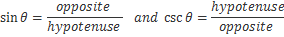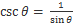Secant is the reciprocal of cosine.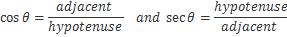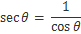Cotangent is the reciprocal of tangent.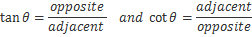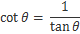When solving right triangles the three main identities are traditionally used. However, the reciprocal functions (secant, cosecant and cotangent) can be helpful in solving trig equations and simplifying trig identities.

 Related Links: Math Trigonometry Inverse Cosine Function (Arccosine) Inverse Sine Function (Arcsine)

To link to this Cotangent, Secant and Cosecant page, copy the following code to your site: